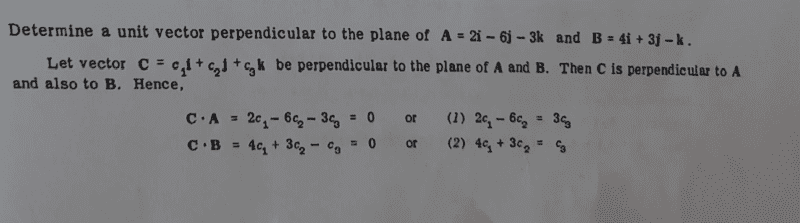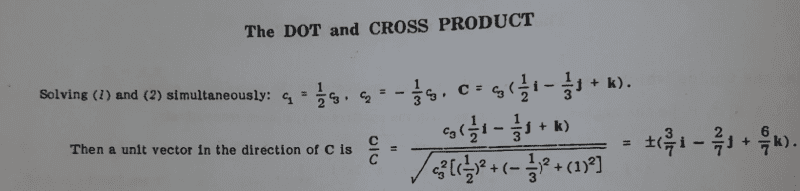# Determine a unit vector perpendicular to the given planes

• chwala

#### chwala

Gold Member
Homework Statement
see attached.
Relevant Equations
vectorsI looked at this question and i wanted to ask if we could also use; ##C## =## c_2 ##(##-\dfrac {3}{2}i## +## j - 3k)## ... cheers

This problem can also be solved by using the approach of cross product ##A×B##...

Last edited:
Homework Statement:: see attached.
Relevant Equations:: vectors

View attachment 297125

View attachment 297126

I looked at this question and i wanted to ask if we could also use; ##C## =## c_2 ##(##-\dfrac {3}{2}i## +## j - 3k)## ... cheers

This problem can also be solved by using the approach of cross product ##A×B##...
They're asking for a unit vector, so you will need to solve for ##c_2##. Doing so will give you exactly the same two vectors as shown in the solution.

I understand that...I just wanted to be certain that we could work with any ##c_1, c_2## or ##c_3##...thanks...working to the given solution is straightforward.

They're asking for a unit vector, so you will need to solve for ##c_2##. Doing so will give you exactly the same two vectors as shown in the solution.
By the way Mark what do you mean by solve for ##c_2?##...in the text they worked with ##c_3## to solution...I am assuming that you mean the same will apply for ##c_2##.
They're asking for a unit vector, so you will need to solve for ##c_2##. Doing so will give you exactly the same two vectors as shown in the solution.

By the way Mark what do you mean by solve for ##c_2##?
Because you wrote "##C = c_2 (-\dfrac {3}{2}i + j - 3k)## and it needs to be a unit vector. There are only two values of ##c_2## that make this a unit vector: one positive and one negative.

What i meant;
The unit vector in the direction of c can also be given by;
##±\dfrac{{-\frac {3}{2}}i+j-3k}{\sqrt \frac{49}{4}}...##

using either ##c_1, c_2## or ##c_3## would work as indicated in my post ##3##.

Last edited:
What i meant;
The unit vector in the direction of c can also be given by;
##±\dfrac{{-\frac {3}{2}}i+j-3k}{\sqrt \frac{49}{4}}##
Yes, but why would you want to write it this way without simplifying it? Dividing by ##\frac{49} 4## is equivalent to multiplying by 2/7. If you do this, you get the same result as shown in the solution.

Yes, but why would you want to write it this way without simplifying it? Dividing by ##\frac{49} 4## is equivalent to multiplying by 2/7. If you do this, you get the same result as shown in the solution.
Yes of course I can simplify that! ...I was just indicating to you on what I meant...we shall end up with same solution...
Cheers Mark. Thanks.

Why don't you check the solution by taking the cross-product of $\vec{A}$ and $\vec{B}$ and normalizing it

I did check and confirmed that the problem can also be solved by using cross- product...where ##A×B=15i-10j+30k## ...

My only query was specific on ##c_1,c_2## and ##c_3##...Cheers

Last edited:
•WWGD
As a side-comment, it seems you're using a book where exercise solutions use round numbers. Notice the scaling was by a nice nimber like 2/7 and not, a.g., ##\sqrt 13 /2 ##.

As a side-comment, it seems you're using a book where exercise solutions use round numbers. Notice the scaling was by a nice nimber like 2/7 and not, a.g., ##\sqrt 13 /2 ##.
That's true...am actually using Schaum's textbook on Vector Analysis.